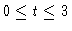## Railway Bogie

exd10
Variables and main parameters:

y1,y3, y5: lateral displacement of front axle, rear axle, bogie frame

y2,y4, y6:yaw displacement of front axle, rear axle, bogie frame

y7: rolling displacement of bogie frame

v: speed of train (bifurcation parameter)

Figure 1
A railway bogie with two axles, schematically

Figure 2
attracting periodic orbit for v=70, y5(t) for, initial vector y1(0)=0.1, yi(0)=0 forFigure 3
transient phase close to the attracting stationary state for v=60, y5(t) for, initial vector y1(0)=0.1, yi(0)=0 forThis document
Figure 1
Figure 2
Figure 3